﻿ Study and Optimization of a Photovoltaic Mill System Functioning on the Course of the Sun
Journal of Material Sciences & Engineering
All submissions of the EM system will be redirected to Online Manuscript Submission System. Authors are requested to submit articles directly to Online Manuscript Submission System of respective journal.
• Research Article
• J Material Sci Eng 2016, Vol 6(1): 309
• DOI: 10.4172/2169-0022.1000309

# Study and Optimization of a Photovoltaic Mill System Functioning on the Course of the Sun

Gaye TA1, Dieng B1*, Sow O2, Mbodji S1 and Sissoko G3
1Department of Physics, UFR Applied Sciences and Information and Communication Technologies, Alioune Diop University, Bambey, Senegal
2University of Thies, Senegal
3Department of Physics, Faculty of Science and Technology, Cheikh Anta Diop University, Dakar, Senegal
*Corresponding Author: Dieng B, Department of Physics, UFR Applied Sciences and Information and Communication Technologies, Alioune Diop University, Bambey, Senegal, Tel: 221339733086, Email: [email protected]

Received Date: Aug 29, 2016 / Accepted Date: Dec 14, 2016 / Published Date: Dec 24, 2016

### Abstract

This communication is centered on the optimization of a photovoltaic mill system performance operating on the sun race. According to the character of the alimented charge which is a DC motor that is running a cereal mill and the meteorological conditions (temperature and light), we can find a big difference between the potential maximal power and the other on which is really transferred to the charge. In order to ameliorate the global output of the system, we use an adapted circuit composed of a boost converter remoted by Pulse Width Modulation (PWM) with a MPPT command (Maximum Power Point Tracking). With the Perturb and Observe ((P&O) algorithm, the MPPT control measure the current and the pressure of the photovoltaic generator field to calculate the power released by this one. According to the result obtained, the MPPT control will adjust the cyclical report of the converter to bring the system to the optimal functioning point. The modelisation then simulation of the system (PV generator, boost converter, Pulse Width Modulation which the MPPT algorithm as for as the direct current charge) are after done with the Matlab/ Simulink software.

Keywords: PV system, PV generator, Boost converter, PWM signal, MPPT control, Perturbation and observation, DC motor, Mill, Matlab/ Simulink

#### Introduction

Currently, solar photovoltaic is no longer limited to light of the bright spots or of powering home devices, but also to power income generating productive equipment, particularly grain mills . Unfortunately, the solar panels offered by manufacturers are still expensive, despite the many efforts by researchers to improve the efficiency of photovoltaic cells . Thus, the use of a solar mill can be considered only in remote areas of the grid. In these circumstances, the use of a photovoltaic generator (PVG) must be subjected to certain requirements:

• The system should be as simple as possible

• The efficiency of the photovoltaic generator must be optimized

• The system must operate automatically and reliably

In this study, we are interested in solar system operating mill "over the sun" to avoid the use of expensive storage batteries and often of mediocre performance. The simplest system, we can have in this case is to directly couple of the PV generator to a DC load (DC motor-mill group for example). But generally, the operating point of the DC load does not match the optimum operating point of the PVG [3-5]. This means that all the energy produced by the PVG is not transmitted to the load.

In order to optimize the system performances, we use a matching circuit composed of a boost converter. This circuit can extract every moment the maximum power available at the terminals of the photovoltaic field and transfer that on the load. This thanks to an order by pulse width modulation (PWM) with a maximum power point tracking (MPPT) strategy.

In this paper, we propose a study of modellization and simulation under Matlab/Simulink of the various components of the PV system and we present the simulation results and discussions for direct connection and the connection optimized by the MPPT technology.

#### Modellization of Photovoltaic System

The system studied is composed of a PV generator, a boost converter, a PWM controller with MPPT technology and a DC motormill group. The following figure shows the block diagram of the overall system (Figure 1). The adaptation circuit is a booster converter inserted between the PV field and DC load and maximizes the power delivered to the load for any level of illumination and temperature, with the MPPT numerical control.

Modellization of PV generator

The power delivered by a photovoltaic cell is not enough to supply a DC load of the size of a motor-mill group. We need to combine multiple cells in series and in parallel to form a module and attain the desired power. Similarly, the interconnection of multiple modules together in series and in parallel provides an even higher power, hence the concept of the photovoltaic generator (PVG) .

If we set Ns, the number of modules in series in a branch and Np, the number of parallel branch, then the electrical diagram of the photovoltaic generator can be represented by Figure 2 . The relationship between the current Ipv (A) and the voltage Vpv (V) to the output of the PVG composed of several modules connected in series and in parallel is modeled in the literature [8,9] by: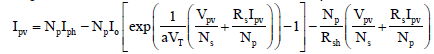(1)

With, Iph: The photocurrent produced by the cell; 0: The saturation current of the diode; Np and Ns: are respectively the number of modules connected in parallel and in series;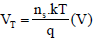is the thermal potential; a: The idealism of the cell factor (a=1.3); ns : is the number of cells connected in series in a module; k=1.38.10 ^ - 23 (J/K): Boltzmann constant; q=1.602.10 ^ - 19© : Charge of the electron; T: is the absolute temperature in kelvin.

The equation of the photocurrent Iph is given by: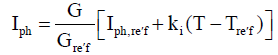(2)

And the current Id absorbed by the diode is: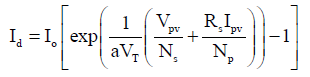With,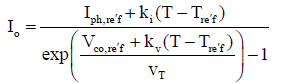(4)

From these equations, we have established the Simulink model of PV generator.

Modellization of boost converter

The boost converter is a DC-DC convertissor voltage elevator inserted between the PV generator and the DC load. Its typical application is to convert the input voltage into a higher output voltage [5,10]. The basic components of a boost converter are: a diode D, a MOSFET transistor Tr which takes two states (closed (u=1) and open (u=0)), an inductor L and an output capacitor Cs. The basic scheme of the boost converter is shown in Figure 3 below.

When the transistor Tr is closed (on mode), the photovoltaic source charging the inductance L, meanwhile, the capacitance Cs maintains the output voltage of the converter using the energy previously stored. When the position of the transistor Trchange (off mode), the DC source and the energy stored in the inductance go together supply the load, resulting in an increase of the output voltage . The modeling of the boost converter can be obtained by applying the fundamental laws governing its operation: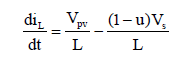(5)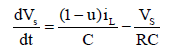(6)

His transformation ratio is, by calling α duty ratio (i.e. ratio of the time during which the transistor is closed, in the period of hash [5,10]: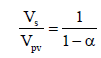(7)

With α between 0 and 1. For a given incident power, the optimum power transferred to the load is maximum for a defined duty cycle.

Modellization of numerical control MPPT "P&O"

In this study, we use the perturbation and observation (P&O) method. This choice is due to the fact that this is a widespread approach in seeking the MPP (Maximum Power Point); in addition it is simple and requires only measurements current and voltage of the PV generator (Ipv and Vpv). It is based on the periodic disturbance of the system by increasing or decreasing the reference voltage (Vréf) or by directly acting on the cyclic ratio α of the converter, and then observing the effect on the output power in order to a possible correction of the duty cycle [5,7,8].

Figure 4 shows the flow chart of the control algorithm "Perturbation and Observation" as it should be implemented in the microprocessor control.

Modellization of PWM control

The reference voltage thus generated by the MPPT control will then be compared with a triangular wave or saw-tooth, for providing a suitable duty cycle. We call this principle the PWM controller (Pulse Width Modulation)  (Figure 5). A comparator to generate at its output a width modulated rectangular voltage (variable duty cycle: PWM signal).

When the voltage Vréf increases (decreases), the duty cycle increases (decreases). The changes in the voltage Vréf induced by given sunlight and temperature, moving the operating point on the characteristic (Ppv-Vpv).

Modellization of the DC motor-mill group

DC Motors are used in the training of electrical machines. In this study it is a grain mill. The motor shaft is connected to the tray who keeping the hammers of mill via a polished-belt system. The block diagram

of such DC load is represented by Figure 6. The DC machine can be modeled through equations electrical, electromagnetic and mechanical . These three groups of equations describe the real operation of the DC motor:

Electrical equation: The equation describes that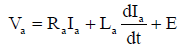(8)

With,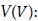The supply voltage of the motor armature;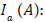The current drawn by the armature;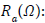The resistance of the armature;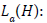> The inductance of the armature; E(V): The electromotive force. The electromotive force (E) is bound by the rotation speed of the motor by the relationship:

E = k.Φ.Ω = ke Ω (9)

With, K is a constant specific to the motor;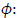The magnetic flux (Wb); ke = k.Φ = E/Ω : constant of electromotive force (V/rad/s); Ω: The rotation speed of motor (rad/s).

Electromagnetic equation: When a current Ia flows in the armature, it appears an electromagnetic torque Tem created by the Lorentz forces acting on the conductors of the armature. This torque is related to the field flux Φ and the current in the armature by the equation .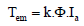(10)

Tem: Electromagnetic torque (N.m)

f the armature has an electromotive force E, while it is traversed by a current of intensity Ia, he received an electromagnetic power Pem(W):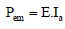(11)

The rotor turns at the angular speed Ω so that the power can also be written: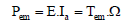(12)

Mechanical equation: The mechanical equation of the electric motor described the ratio between the moment of inertia (kg.m²), the rotation speed Ω (rad/s) and the torque T (N.m). By equating the motor torque to the electromagnetic torque (true to a constant: friction torque), this equation is given in  by: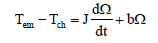(13)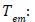The electromagnetic torque (N.m);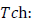The load torque (N.m); J: The total moment of inertia (motor + driven load) (kg.m²); Ω: The rotation speed of the motor shaft (rad/se );b: The coefficient of friction proportional at the rotation speed.

#### Simulation Results and Discussions

Simulation results of PVG

The results obtained after simulation of the PV generator are shown in the figure below, it represents the current-voltage and power-voltage characteristic of the PV generator under standard test conditions (G=1000 W/m², T=25°C) (Figure 7). These results show the nonlinearity characteristics of the PV generator. Indeed, on the curve I-V (or PV), there's a point where the power delivered by the PV generator is maximum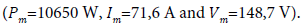To know the effect of the temperature on the performance of the PV system, we are presented in Figure 8 the current-voltage and powervoltage characteristic for a constant sunshine level (Gréf=1000 W/m²) and for different temperatures.

Figure 7: Simulation results of the characteristic I-V and P-V of PV generator, for G=1000 W/mÂ² and T=25Â°C.

Figure 8: Simulation results of the influence of temperature on the characteristic I-V and P-V of the PV generator to Gref=1000 W/mÂ².

We can see that the increase of temperature causes a small drop in the power available at the terminals of the PV generator. For to know the effect of solar radiation on the I-V and P-V characteristics of the PV generator, we set the temperature at Tréf=25°C, and vary the amount of sunlight from 400 to 1000 W/m². We get the following results (Figure 9). These results show that the current produced by the PV generator is highly dependent on solar radiation, but against the voltage varies slightly. Then the maximum power point (MPP) of PV generator varies with decreasing sunlight.

Figure 9: Simulation results of the influence of sunlight on the characteristic I-V and P-V of the PV generator to Tref=25Â°C.

Simulation results of the boost converter

The training Matlab/Simulink of the boost converter has to have very interesting results. Figure 10 shows the signal applied to the gate of the transistor corresponds to a switching frequency set at 50%. The below Figure 11 shows the input and output voltage of the boost converter after simulation. These results show that the voltage at the output of the converter is greater than the input. So the DCDC converter performs its role properly.

Simulation results of direct coupling PVG - DC load

Simulation results for direct connection are shown in Figure 12, he shows the current-voltage characteristic of the PVG superimposed on that of the load. These results show that the operating point in direct coupling does not match the maximum power point of the PV generator. We can observe that the PV generator is poorly operated and does not provide the maximum potential power. The difference being wasted as heat dissipated in the PVG. This can be explained by the fact that the nominal operating voltage of the load is different from the optimum voltage (Vapt) of PVG. With the measured values of the PVG characteristics (T=25°C and G=1000 W/m²). We have: Im=71,6 A; Vm=148,7 V; Pm=10650 W. Direct connection was simulated output power: Is=51,4 A; Vs=161, 9 V; Ps=8324 W. In this case, there is an efficiency of: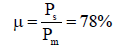Simulation results of system optimized by the MPPT technology (P&O)

The results obtained are represented in Figure 13 which gives the I-V characteristic of the PVG superimposed on the I-V characteristic of the load. These results show that the operating point of the system converges to the maximum power point of the PV generator. With the measured values of the PVG characteristics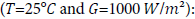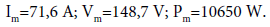With the optimized system, we obtained by simulation:

Is=69,14 A; Vs=152,2 V; Ps=10488 W.

It will in this case an efficiency of: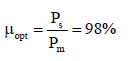#### Conclusion

In this paper, we modelled and simulated all the components of a solar mill system: PV generator, booster converter, MPPT controls "Perturbation and Observation" and load (DC motor-mill group). Also, the simulation results and discussions for direct connection PVG-load and indirect connection controlled by a converter are presented. From these results, we note that the performances of PVG degrade with fluctuations in weather conditions (temperature and sunlight). The boost converter and the MPPT control (P&O) properly perform their role. The boost converter provides an output voltage higher than the input voltage. And the MPPT control adjusts the PV generator to the load: there's a maximum transfer of the available power to the terminals of the PV generator.

#### References

Citation: Gaye TA, Dieng B, Sow O, Mbodji S, Sissoko G (2016) Study and Optimization of a Photovoltaic Mill System Functioning on the Course of the Sun. J Material Sci Eng 6: 309. Doi: 10.4172/2169-0022.1000309

Copyright: © 2016 Gaye TA, et al. This is an open-access article distributed under the terms of the Creative Commons Attribution License, which permits unrestricted use, distribution, and reproduction in any medium, provided the original author and source are credited.

Select your language of interest to view the total content in your interested language

##### Recommended Journals
Viewmore
• ###### 24th International Conference on Advanced Materials & Nanotechnology

September 19-20, 2019 Brussels, Belgium

• ###### World Congress on Carbon and Advanced Energy Materials

September 23-24, 2019 Hong Kong, Thailand

• ###### 21st International Conference on Advanced Materials Science & Nano Technology

September 26-27, 2019 Dubai, UAE

• ###### 5th International Conference on Crystallography & Novel Materials

November 18-19, 2019 Helsinki, Finland

• ###### 10th International Conference on Biopolymers and Polymer Sciences

November 18-19, 2019 Helsinki, Finland

Viewmore
###### Article Usage
• Total views: 1437
• [From(publication date): 0-2017 - Aug 24, 2019]
• Breakdown by view type
• HTML page views: 1346
• PDF downloads: 91

## Post your commentCan't read the image? click here to refresh
###### Peer Reviewed Journals

Make the best use of Scientific Research and information from our 700 + peer reviewed, Open Access Journals

International Conferences 2019-20

Meet Inspiring Speakers and Experts at our 3000+ Global Annual Meetings

Top